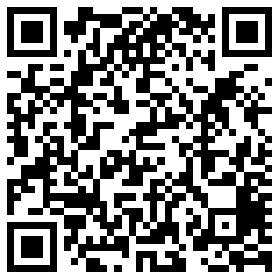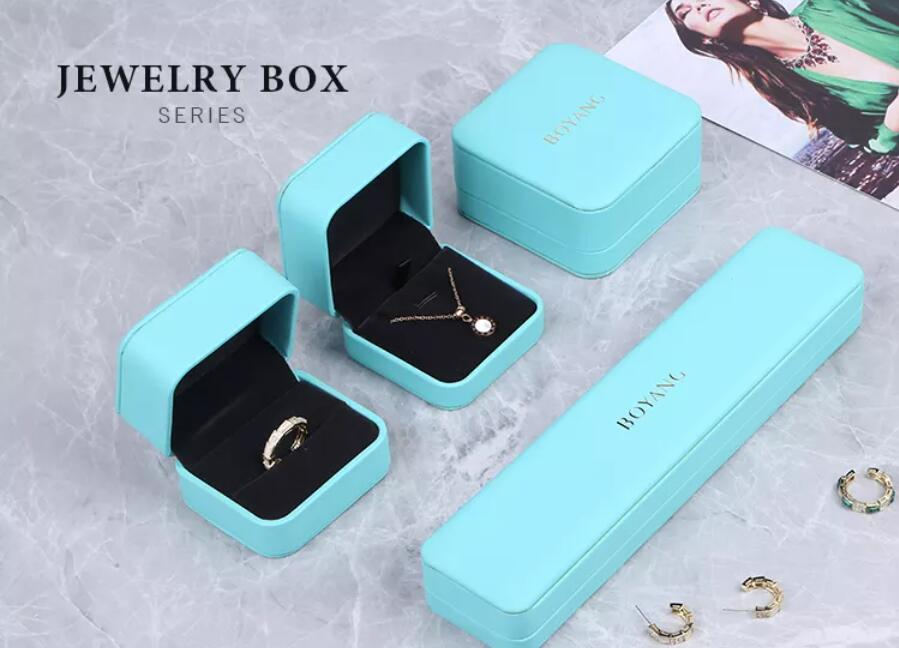•# How to measure the volume of the jewelry packaging box?

• 615
• Jimmy at
• September 20, 2023

When customizing jewelry packaging boxes, you need to consider the volume of the jewelry packaging box for better transportation and storage. Measuring the volume of a jewelry box is a relatively simple task that can be accomplished using basic geometric shapes. You'll need a few tools: a ruler or tape measure, a calculator, and paper and pen to record measurements and calculations.

Measuring the volume of jewelry packaging boxes requires knowing three dimensions. The first step to creating the perfect package is to measure three dimensions on the inside of the box, not the outside, using a ruler or tape measure and round each result to the nearest whole inch. But to do this we must know three dimensions: length, width, and depth.Ruler or tape measure
calculator
pen and paper

2. Clean the box:

Remove any jewelry or padding from the box to ensure accurate measurements.

3. Measure length, width, and height:

Use a ruler or measuring tape to measure the length (L), width (W), and height (H) of your jewelry box. Make sure to measure from the inside of the box so you can accurately calculate the volume.
All three dimensions are measured using the same unit of measurement (such as inches or centimeters).

4. Record the measurement results:

Write the length, width, and height measurements on a piece of paper.

5. Calculate volume:

To calculate the volume (V) of a jewelry box, use the formula for the volume of a cuboid (box):
V = length x width x height
Substitute the measurements for L, W, and H into the formula.

6. Perform calculations:

Use a calculator to perform multiplication and find the volume.

7. Calculate the volume value:

Express the volume in the appropriate units (cubic inches, cubic centimeters, etc.) based on your initial measurements.
For example, if you measured a jewelry box and found that it was 6 inches long, 4 inches wide, and 2 inches tall, you would calculate the volume as follows:
V = 6 inches x 4 inches x 2 inches = 48 cubic inches
Well, the volume of the jewelry box is 48 cubic inches.

This method is suitable for rectangular or cuboid-shaped jewelry packaging boxes. If the box has an irregular shape, you may want to break it into smaller regular shapes (for example, a cuboid or a cube) and calculate the volume of each part separately, then add them together to get the total volume.

Technical Support: Magic Lamp